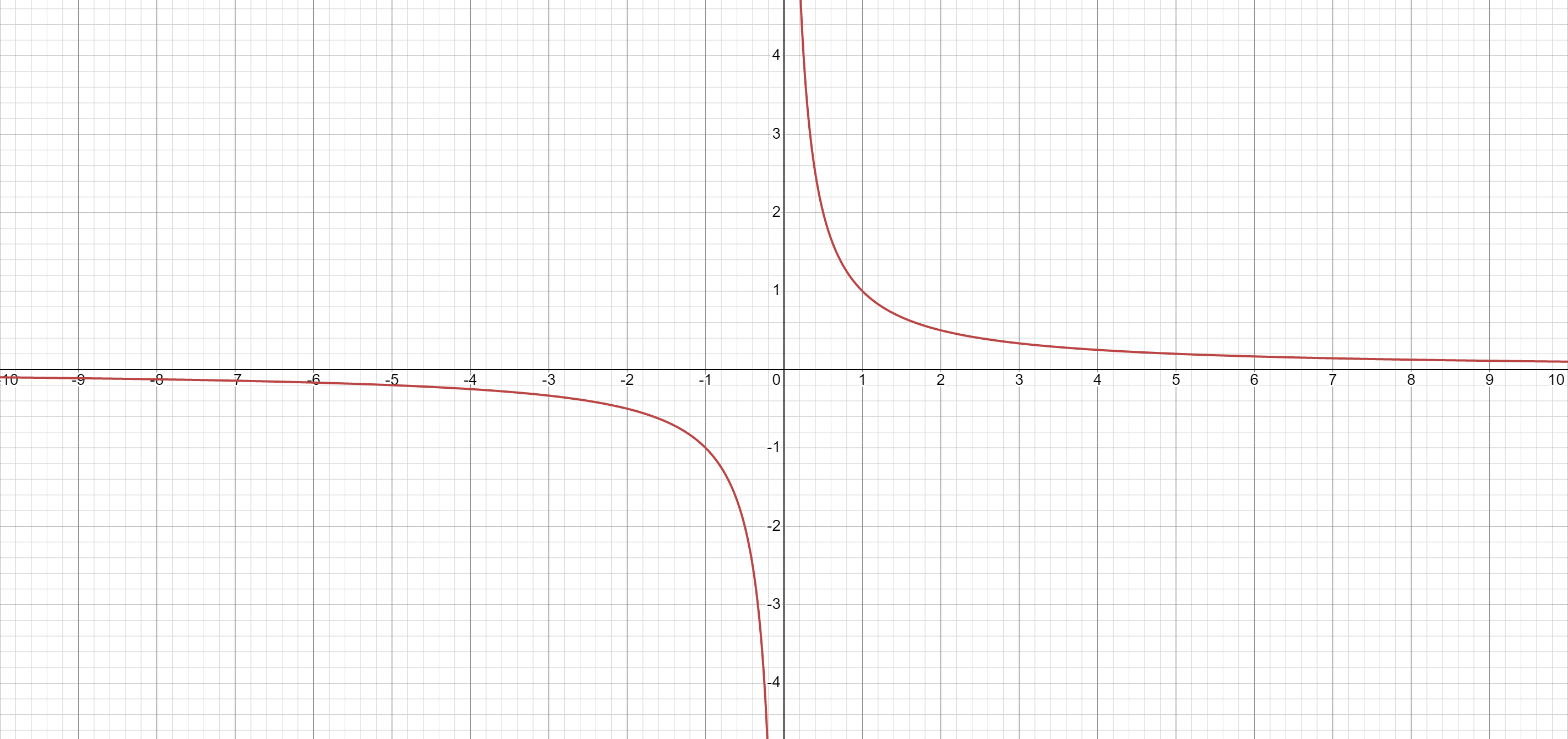2022-01-19

find and sketch the domain of the function f(x,y)= x/x^2+y^2xleb123

Expert

$f\left(x,y\right)=\frac{x}{{x}^{2}}+{y}^{2}$

Cancel the common factor of $x$ and ${x}^{2}$.

Raise $x$ to the power of $1$.

$f\left(x,y\right)=\frac{{x}^{1}}{{x}^{2}}+{y}^{2}$

Factor $x$ out of ${x}^{1}$.

$f\left(x,y\right)=\frac{x\cdot 1}{{x}^{2}}+{y}^{2}$

Cancel the common factors.

$f\left(x,y\right)=\frac{1}{{x}^{}}+{y}^{2}$

Subtract ${y}^{2}$ from both sides of the equation.

$f\left(x,y\right)-{y}^{2}=\frac{1}{{x}^{}}$

Set the denominator in $\frac{1}{{x}^{}}$ equal to 0 to find where the expression is undefined.

$x=0$

The domain is all values of x that make the expression defined.

Interval Notation:

$\left(-\infty ,0\right)\cup \left(0,\infty \right)$

Set-Builder Notation:

$\left\{x|x\ne 0\right\}$Do you have a similar question?